Test: Functions Of One,Two Or Three Real Variables -3

# Test: Functions Of One,Two Or Three Real Variables -3

Test Description

## 20 Questions MCQ Test Topic-wise Tests & Solved Examples for IIT JAM Mathematics | Test: Functions Of One,Two Or Three Real Variables -3

Test: Functions Of One,Two Or Three Real Variables -3 for IIT JAM 2023 is part of Topic-wise Tests & Solved Examples for IIT JAM Mathematics preparation. The Test: Functions Of One,Two Or Three Real Variables -3 questions and answers have been prepared according to the IIT JAM exam syllabus.The Test: Functions Of One,Two Or Three Real Variables -3 MCQs are made for IIT JAM 2023 Exam. Find important definitions, questions, notes, meanings, examples, exercises, MCQs and online tests for Test: Functions Of One,Two Or Three Real Variables -3 below.
Solutions of Test: Functions Of One,Two Or Three Real Variables -3 questions in English are available as part of our Topic-wise Tests & Solved Examples for IIT JAM Mathematics for IIT JAM & Test: Functions Of One,Two Or Three Real Variables -3 solutions in Hindi for Topic-wise Tests & Solved Examples for IIT JAM Mathematics course. Download more important topics, notes, lectures and mock test series for IIT JAM Exam by signing up for free. Attempt Test: Functions Of One,Two Or Three Real Variables -3 | 20 questions in 60 minutes | Mock test for IIT JAM preparation | Free important questions MCQ to study Topic-wise Tests & Solved Examples for IIT JAM Mathematics for IIT JAM Exam | Download free PDF with solutions
 1 Crore+ students have signed up on EduRev. Have you?
Test: Functions Of One,Two Or Three Real Variables -3 - Question 1

### The function f(x) = - 2x3 - 9x2 - 12x + 1 is an increasing function in the interval

Detailed Solution for Test: Functions Of One,Two Or Three Real Variables -3 - Question 1

Given that
f(x) = - 2x3 - 9x2 - 12x + 1
- + -
- 2 - 1
implies f'(x) = - 6x2 - 18x - 12
= -6[x2 + 3x + 2]
​= - 6[(x + l ) ( x + 2)]
implies f'(x) > 0, V x ∈ (-2, -1)
Hence, f(x) is increasing in the interval (-2, -1)

Test: Functions Of One,Two Or Three Real Variables -3 - Question 2

### If f(x) is real-valued function defined on [0, ∞] such that f(0) = 0 and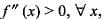then the function h(x)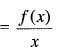is

Detailed Solution for Test: Functions Of One,Two Or Three Real Variables -3 - Question 2

Given that,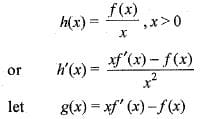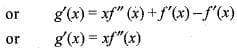Since, f''(x) > 0 and x > 0, therefore g'(x) > 0,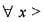0 => g (x) is strictly increasing function in [0, ∞]
since, h'(x)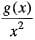=> h'(x) > 0,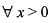or h (x) is increasing in [0, ∞]

Test: Functions Of One,Two Or Three Real Variables -3 - Question 3

### f(x) = x6 - x - 1, x ∈ [1, 2], consider the following statements (i) f is increasing on [1, 2] (ii) f has root in [1, 2] (iii) f is decreasing in [1, 2] (iv) f has no root in [1 ,2] Q. which of the above is/are correct?

Detailed Solution for Test: Functions Of One,Two Or Three Real Variables -3 - Question 3

Given that f(x) = x6 - x - 1
or f'(x) = 6x5- 1
or f'(x) > 0,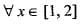Clearly, f(x) is continuous in the interval [1, 2]
Such that f(1) f(2) < 0, therefore , f(x ) has atleast one root between [1, 2]

Test: Functions Of One,Two Or Three Real Variables -3 - Question 4

The value of ‘C’ of Lagrange’s mean value theorem for f( x ) = x (x - 1) in [1 ,2] is given by

Detailed Solution for Test: Functions Of One,Two Or Three Real Variables -3 - Question 4

Clearly f(x) = x2 - x is continuous in the interval [1, 2] and differentiable in the open interval [1,2]. therefore,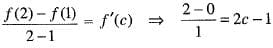or 2 = 2c - 1
or c = 3/2

Test: Functions Of One,Two Or Three Real Variables -3 - Question 5

If f(x) =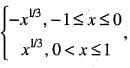then

Detailed Solution for Test: Functions Of One,Two Or Three Real Variables -3 - Question 5

Given that,
f(x) =Clearly the given function is not differentiable at x = 0. Therefore, Roll’s theorem does not apply to f in [-1,1]

Test: Functions Of One,Two Or Three Real Variables -3 - Question 6

Let f(x, y) =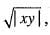then

Detailed Solution for Test: Functions Of One,Two Or Three Real Variables -3 - Question 6

We are given that
f(x,y) =Let us take ε > 0 and (x, y) ≠ (0, 0). Consider
|f(x,y) - 0| =Let x = r cos θ, y = r sin θ
Therefore,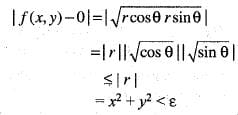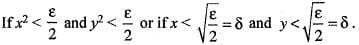Hence for given ε > 0, there exists δ > 0 such that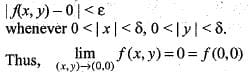Therefore, f(x, y) is continuous at (0, 0). Hence option (a) is correct.
Next,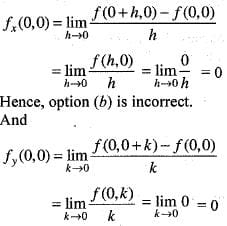Hence, option (c) is incorrect.

Test: Functions Of One,Two Or Three Real Variables -3 - Question 7

Let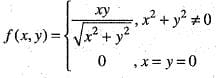Detailed Solution for Test: Functions Of One,Two Or Three Real Variables -3 - Question 7

We are given that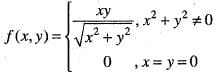Let us take ε > 0 and x2 + y2 ≠ 0.
Now consider,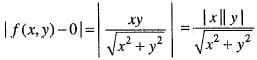We know that,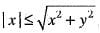or equivalently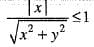Thus,
| f(x ,y )-0 | ≤ | y | = 0- |x| + 1 • |y|
take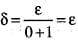Therefore, for given ε > 0, there exists δ > 0 such that
|f(x, y) - 0 | < ε. whenever 0<|x|<δ,
0< |y |< δ
Hence,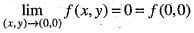Thus, f(x,y) is continuous at (0, 0).
Hence, option (a) is correct.
Now,
f(0 + h ,0 + k) - f(0,0) = f(h , k) - f(*0,0)
= 0 • h + 0 • k +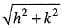•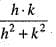So that A = 0, B = 0 which does not depends on h and k and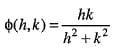Now approaching along h = mk, we get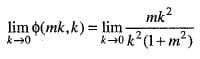which depends on m. Therefore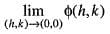does not exists. Hence, f(x, y) is not differentiable at origin. Hence, option (b) is incorrect.
Next,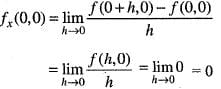Hence, option (c) is incorrect.
And,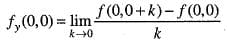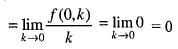Hence, option (d) is incorrect.

Test: Functions Of One,Two Or Three Real Variables -3 - Question 8

Let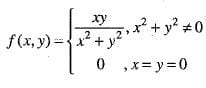then

Detailed Solution for Test: Functions Of One,Two Or Three Real Variables -3 - Question 8

Solution :- Consider an approach along the line y=x; then f(x,y)=x^2/(x^2+x^2) = 1/2

for all x≠0. On the other hand, if we approach (0,0) along the line y=2x

f(x,y)=(2x)^2/[x^2+4x^2]

=2/5

So there are two different paths toward the origin, each giving a different limit. Hence, the limit does not exist.

Or more simply, approach along y=0

Therefore , f(x, y) is not differentiable at origin

Test: Functions Of One,Two Or Three Real Variables -3 - Question 9

Let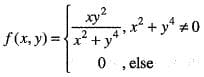Then, the directional derivative at c = (0, 0) in the direction u = (a1, a2) is

Detailed Solution for Test: Functions Of One,Two Or Three Real Variables -3 - Question 9

We are given that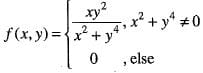Then, the directional derivative at c = (0, 0) in the direction u(a1, a2) is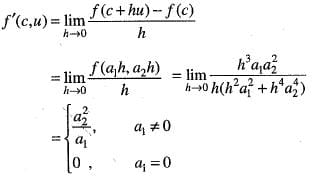and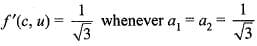Hence, option (c) is correct.

Test: Functions Of One,Two Or Three Real Variables -3 - Question 10

Let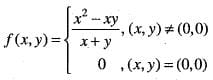Then,

Detailed Solution for Test: Functions Of One,Two Or Three Real Variables -3 - Question 10

We are given that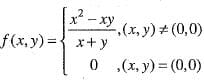Now,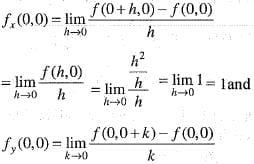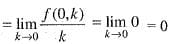Hence, option (b) is correct,

Test: Functions Of One,Two Or Three Real Variables -3 - Question 11

The derivative of the function f(x) = x2m is

Detailed Solution for Test: Functions Of One,Two Or Three Real Variables -3 - Question 11

Here f(x) = x2m is an even function f'(x) = 2mx2m-1 is an odd function.

Test: Functions Of One,Two Or Three Real Variables -3 - Question 12

Let f(x) = | sin πx |,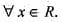then

Detailed Solution for Test: Functions Of One,Two Or Three Real Variables -3 - Question 12

The graph of the function f(x) = | sinπx | is y =f(x)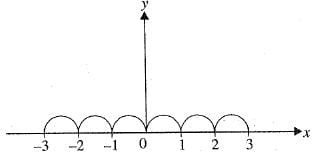clearly, the graph have sharp edges at the points 0, ±1, ± 2, ±3, .. therefore function is continuous everywhere but not differentiable everywhere except at integral values of x.

Test: Functions Of One,Two Or Three Real Variables -3 - Question 13

If f'(a) exists, then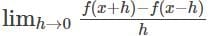is equal to

Detailed Solution for Test: Functions Of One,Two Or Three Real Variables -3 - Question 13

Since, f'(a) exists, then, we have
Lf'(a) = f '(a) and Rf'(a) = f'(a)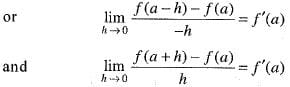Adding these two, we get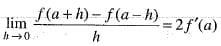Test: Functions Of One,Two Or Three Real Variables -3 - Question 14

A function is said to be ______________ if and only if f(a) = f(b) implies that a = b for all a and b in the domain of f.

Detailed Solution for Test: Functions Of One,Two Or Three Real Variables -3 - Question 14

A function is one-to-one if and only if f(a) ≠ f(b) whenever a ≠ b.

Test: Functions Of One,Two Or Three Real Variables -3 - Question 15

The continuous function f : R -->R defined by f(x) = (x+ 1)2011 is

Detailed Solution for Test: Functions Of One,Two Or Three Real Variables -3 - Question 15

Since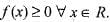therefore is not onto and f(- 1) = f(1) , therefore, f(x) is not one-one.

Test: Functions Of One,Two Or Three Real Variables -3 - Question 16

Let
f(x, y) = 2x2 - xy + 2y2 Then at (1, 2)

Detailed Solution for Test: Functions Of One,Two Or Three Real Variables -3 - Question 16

We are given that
f(x, y) = 2x2 - xy + 2y2
then,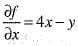Hence,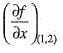= 4 x 1 - 2 = 2
and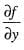= - x + 4y
Hence,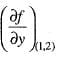= - l + 4x 2 = 7
Thus,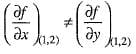Hence, option (d) is correct.

Test: Functions Of One,Two Or Three Real Variables -3 - Question 17

Let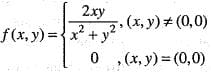then,

Detailed Solution for Test: Functions Of One,Two Or Three Real Variables -3 - Question 17

We are given that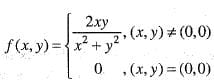Now,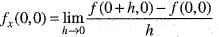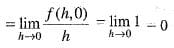and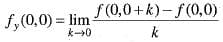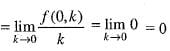Hence, option (b) is correct.

Test: Functions Of One,Two Or Three Real Variables -3 - Question 18

If any four numbers are selected and they are multiplied, then the probability that the last digit will be 1, 3, 5 or 7 is

Detailed Solution for Test: Functions Of One,Two Or Three Real Variables -3 - Question 18

The total number of digits in any number at the units place is 10.
Therefore, n (S) = 10
If the last digit is 1, 3, 5 or 7, then it is necessary that the last digit in each number must be 1, 3, 5 or 7.
Therefore, n (A) = 4
P (A) = 4 / 10 = 2 / 5
Hence, the required probability is (2 / 5)4 = 16 / 625.

Test: Functions Of One,Two Or Three Real Variables -3 - Question 19

Let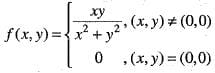Then,

Test: Functions Of One,Two Or Three Real Variables -3 - Question 20

Let
f(x,y) = x3 + y3- 63 (x + y) + 12xy,
then

## Topic-wise Tests & Solved Examples for IIT JAM Mathematics

27 docs|150 tests
 Use Code STAYHOME200 and get INR 200 additional OFF Use Coupon Code
Information about Test: Functions Of One,Two Or Three Real Variables -3 Page
In this test you can find the Exam questions for Test: Functions Of One,Two Or Three Real Variables -3 solved & explained in the simplest way possible. Besides giving Questions and answers for Test: Functions Of One,Two Or Three Real Variables -3, EduRev gives you an ample number of Online tests for practice

## Topic-wise Tests & Solved Examples for IIT JAM Mathematics

27 docs|150 tests